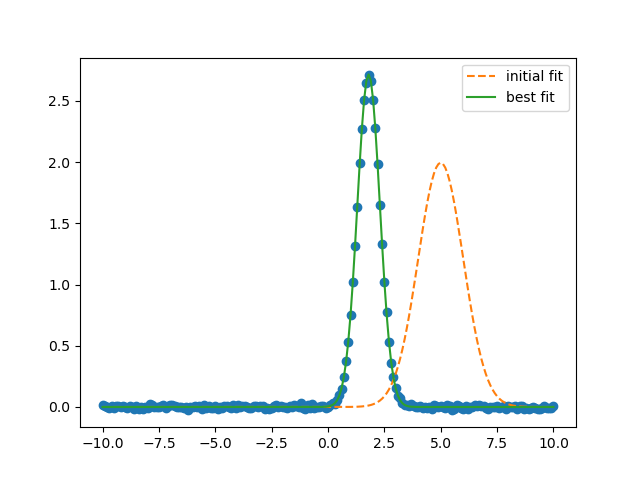# Using an ExpressionModel¶

ExpressionModels allow a model to be built from a user-supplied expression. See: https://lmfit.github.io/lmfit-py/builtin_models.html#user-defined-models

import matplotlib.pyplot as plt
import numpy as np

from lmfit.models import ExpressionModel


Generate synthetic data for the user-supplied model:

x = np.linspace(-10, 10, 201)
amp, cen, wid = 3.4, 1.8, 0.5

y = amp * np.exp(-(x-cen)**2 / (2*wid**2)) / (np.sqrt(2*np.pi)*wid)
y = y + np.random.normal(size=x.size, scale=0.01)


Define the ExpressionModel and perform the fit:

gmod = ExpressionModel("amp * exp(-(x-cen)**2 /(2*wid**2))/(sqrt(2*pi)*wid)")
result = gmod.fit(y, x=x, amp=5, cen=5, wid=1)


this results in the following output:

print(result.fit_report())

plt.plot(x, y, 'bo')
plt.plot(x, result.init_fit, 'k--', label='initial fit')
plt.plot(x, result.best_fit, 'r-', label='best fit')
plt.legend(loc='best')
plt.show()Out:

[[Model]]
Model(_eval)
[[Fit Statistics]]
# fitting method   = leastsq
# function evals   = 56
# data points      = 201
# variables        = 3
chi-square         = 0.01825874
reduced chi-square = 9.2216e-05
Akaike info crit   = -1864.58964
Bayesian info crit = -1854.67973
[[Variables]]
amp:  3.40013669 +/- 0.00494962 (0.15%) (init = 5)
cen:  1.80087014 +/- 8.3982e-04 (0.05%) (init = 5)
wid:  0.49962144 +/- 8.3982e-04 (0.17%) (init = 1)
[[Correlations]] (unreported correlations are < 0.100)
C(amp, wid) =  0.577


Total running time of the script: ( 0 minutes 0.283 seconds)

Gallery generated by Sphinx-Gallery